# Electronics and Communication Engineering - Electromagnetic Field Theory

41.

A transmission line is feeding 1 watt of power to a horn antenna having a gain of 10 dB. The antenna is matched to the transmission line. The total power radiated by the horn antenna into the free space is

 A. 10 watts B. 1 watt C. 0.1 watt D. 0.01 watt

Answer: Option A

Explanation: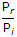= 101Pr = 10 watt.

42.

A parallel polarized wave is incident from air into paraffin having relative permittivity 3, the value of its Brewster angle is

 A. 0° B. 30° C. 45° D. 60°

Answer: Option D

Explanation:

Brewster angle θ = tan-1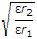.

43.

In a conductor which of the following relations hold good?

 A. ∇ x D = r B. ∇ . D = r C. ∇ x D = 0 D. ∇ . D = 0

Answer: Option C

Explanation:

Inside a conductor free charge is zero, hence, ∇ x D = 0.

44.

The radiation resistance of a λ/16 wire dipole in free space will be nearly

 A. 1 Ω B. 3 Ω C. 13 Ω D. 30 Ω

Answer: Option B

Explanation:

Rr = 80p2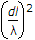.

45.

Two identical antennas are placed in the θ = p/2 plane as shown in the figure. The elements have equal amplitude excitation with 180° polarity difference, operating of the far-zone resultant electric field strength normalized with that of a single element, both computed for φ = 0, is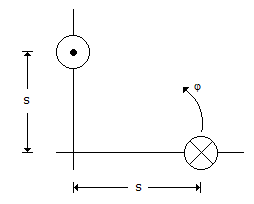A.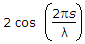B.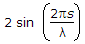C.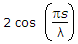D.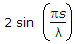Answer: Option D

Explanation:

Normalised array factor = n cos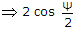where φ is the phase difference.φ = (βd sin θ cos φ + θ1)

and θ = 90°, φ = 45°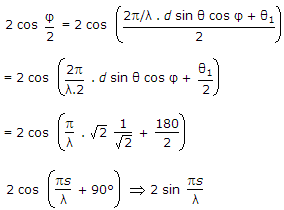#### Current Affairs 2021

Interview Questions and Answers